Showing posts with label Engineering Mechanics. Show all posts
Showing posts with label Engineering Mechanics. Show all posts

### Engineering Mechanics

Basics of engineering mechanics statics and dynamics course with all fundamentals and basic concepts of the subject. Below is complete outline of the subject as taught in mechanical engineering undergraduate course. It is compulsory for all mechanical engineers to revise basic concepts of engineering mechanics syllabus and other courses to stay updated with knowledge of the subjects and for a better performance during interviews. Engineering mechanics is a core subject in mechanical engineering degree taught during junior years of undergraduate degree because this is where engineering starts from. It has been observed that engineering students fail to give appropriate answers to technical questions during job interviews. This short introduction to all topics of engineering mechanics will help you revise all basic concepts.

### Engineering Mechanics - Introduction

The Engineering Mechanics is that branch of Engineering-science which deals with the principles of mechanics along with their applications to engineering problems. It is sub-divided into the following two main groups:

(a) Statics, and (b) Dynamics

The Statics is that branch of Engineering Mechanics which deals with the forces and their effects, while acting upon the bodies at rest.

The Dynamics is that branch of Engineering Mechanics which deals with the forces and their effects, while acting upon the bodies in motion. It is further sub-divided into the following two branches:

(i) Kinetics, and (ii) Kinematics

The Kinetics is that branch of Dynamics, which deals with the bodies in motion due to the application of forces.

The Kinematics is that branch of Dynamics which deals with the bodies in motion without taking into account the forces which are responsible for the motion.

### Force - Basic Definition

Force may be defined as an agent which produces or tends to produce, destroy or tends to destroy the motion of a body. A force while acting on a body may

(a) change the motion of a body,
(b) retard the motion of a body,
(c) balance the forces already acting on a body, and
(d) give rise to the internal stresses in a body.

In order to determine the effects of a force acting on a body, we must know the following characteristics of a force:

(i) The magnitude of the force,
(ii) The line of action of the force,
(iii) The nature of the force, i.e. push or pull, and
(iv) The point at which the force is acting.

In M. K. S. system of units, the magnitude of the force is expressed in kilogram-force (briefly written as kgf) and in S.I. system of units, the force is expressed in newtons (briefly written as N). It may be noted that

1 kgf = 9.81 N

### Resultant Force

Resultant Force - It is a single force which produces the same effect as produced by all the given forces acting on a body. The resultant force may be determined by the following three laws of forces :

1. Parallelogram law of forces. It states that if two forces, acting simultaneously on a particle, be represented in magnitude and direction by the two adjacent sides of a parallelogram, then their resultant may be represented in magnitude and direction by the diagonal of a parallelogram which passes through their points of intersection.

For example, let us consider two forces P and Q acting at angle θ at point O as shown in Fig. 1.1. The resultant is given by,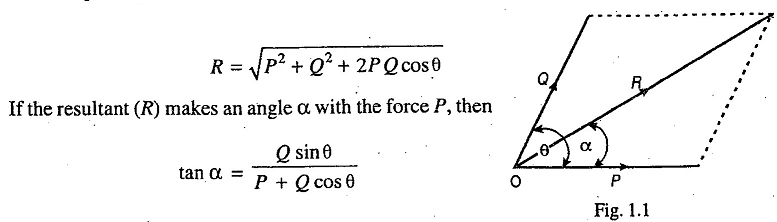2. Triangle law of forces. It states that if two forces, acting simultaneously on a particle, be represented in magnitude and direction by the two sides of a triangle taken in order, then their resultant may be represented in magnitude and direction by the third side of the triangle taken in opposite order.

3. Polygon law of forces. It states that if a number of forces, acting simultaneously on a particle, be represented in magnitude and direction by sides of a polygon taken in order, then their resultant is represented in magnitude and direction by the closing side of the polygon taken in opposite order.

Notes :
1. The resultant of more than two intersecting forces may be found out by resolving all the forces horizontally and vertically. In such cases, resultant of the forces is given by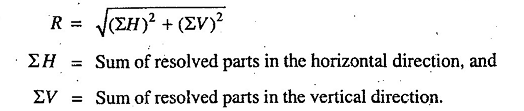If the resultant (R) makes an angle α with the horizontal, then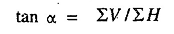2. If the resultant of a number of forces, acting on a particle, is zero then the particle will be in equilibrium. Such a set of forces, whose resultant is zero, are known as equilibrium forces. The force, which brings the set of forces in equilibrium is called an equilibriant. It is equal to the resultant force in magnitude but opposite in direction.

3. A number of forces acting on a particle will be in equilibrium when: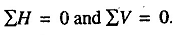### System of Forces

When two or more than two forces act on a body, they are said to form a system of forces. Following are the various system of forces:
1. Coplanar forces. The forces, whose lines of action lie on the same plane are known as coplanar forces.
2. Concurrent forces. The forces, which meet at one point, are known as concurrent forces.
3. Coplanar concurrent forces. The forces, which meet at one point and their lines of action also lie on the same plane, are called coplanar concurrent forces.
4. Coplanar non-concurrent forces. The forces, which do not meet at one point but their lines action lie on the same plane, are known as coplanar non-concurrent forces.
5. Non-coplanar concurrent forces. The forces, which meet at one point but their lines of action do not lie on the same plane are known as non-coplanar concurrent forces.
6. Non-coplanar non-concurrent forces. The forces, which do not meet at one point and their lines of actions do not lie on the same plane are called non-coplanar non-concurrent forces.

### Lami's Theorem

Lami's Theorem states that if three coplaner forces acting at a point be in equilibrium, then each force is proportional to the sine of the angle between the other two forces. Mathematically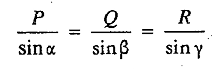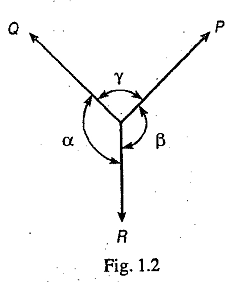where P, Q and R are the three forces and α, β and γ are the angles as shown in Fig 1.2.